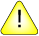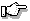This version of this document is no longer maintained. For the latest documentation, see http://www.qnx.com/developers/docs.

sin(), sinf()

Calculate the sine of an angle

Synopsis:

#include <math.h>

double sin( double x );

float sinf( float x );

Arguments:

x
The angle, in radians, for which you want to compute the sine.

Library:

libm

Use the -l m option to qcc to link against this library.

Description:

The sin() and sinf() functions compute the sine (specified in radians) of x. An argument with a large magnitude may yield a result with little or no significance.

Returns:

The sine value.If an error occurs, these functions return 0, but this is also a valid mathematical result. If you want to check for errors, set errno to 0, call the function, and then check errno again. These functions don't change errno if no errors occurred.

Examples:

#include <stdio.h>
#include <math.h>
#include <stdlib.h>

int main( void )
{
printf( "%f\n", sin(.5) );

return( EXIT_SUCCESS );
}

produces the output:

0.479426

Classification:

Safety:
Cancellation point No
Interrupt handler No
Signal handler No